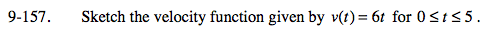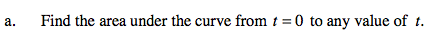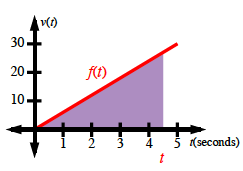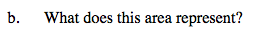### Home > PC > Chapter 9 > Lesson 9.3.5 > Problem9-157

9-157.
1.Sketch the velocity function given by v(t) = 6t for 0 ≤ t ≤ 5. Homework Help ✎

1. Find the area under the curve from t = 0 to any value of t.

2. What does this area represent?$\text{Area} = \frac{(\text{base})(\text{height})}{2}$

$\text{Area} = \frac{(t)(f(t))}{2}$

$\text{Area} = \frac{(t)(6t)}{2}$

Area = 3t2It represents distance traveled.